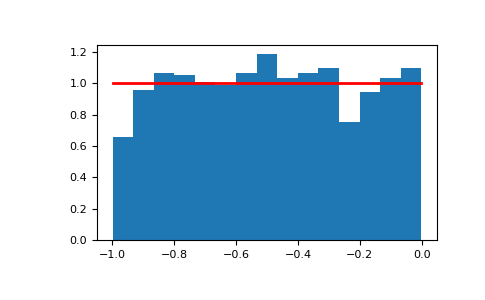# numpy.random.Generator.uniform¶

method

random.Generator.uniform(low=0.0, high=1.0, size=None)

Draw samples from a uniform distribution.

Samples are uniformly distributed over the half-open interval [low, high) (includes low, but excludes high). In other words, any value within the given interval is equally likely to be drawn by uniform.

Parameters
lowfloat or array_like of floats, optional

Lower boundary of the output interval. All values generated will be greater than or equal to low. The default value is 0.

highfloat or array_like of floats

Upper boundary of the output interval. All values generated will be less than high. high - low must be non-negative. The default value is 1.0.

sizeint or tuple of ints, optional

Output shape. If the given shape is, e.g., (m, n, k), then m * n * k samples are drawn. If size is None (default), a single value is returned if low and high are both scalars. Otherwise, np.broadcast(low, high).size samples are drawn.

Returns
outndarray or scalar

Drawn samples from the parameterized uniform distribution.

integers

Discrete uniform distribution, yielding integers.

random

Floats uniformly distributed over [0, 1).

Notes

The probability density function of the uniform distribution is

$p(x) = \frac{1}{b - a}$

anywhere within the interval [a, b), and zero elsewhere.

When high == low, values of low will be returned.

Examples

Draw samples from the distribution:

>>> s = np.random.default_rng().uniform(-1,0,1000)


All values are within the given interval:

>>> np.all(s >= -1)
True
>>> np.all(s < 0)
True


Display the histogram of the samples, along with the probability density function:

>>> import matplotlib.pyplot as plt
>>> count, bins, ignored = plt.hist(s, 15, density=True)
>>> plt.plot(bins, np.ones_like(bins), linewidth=2, color='r')
>>> plt.show()